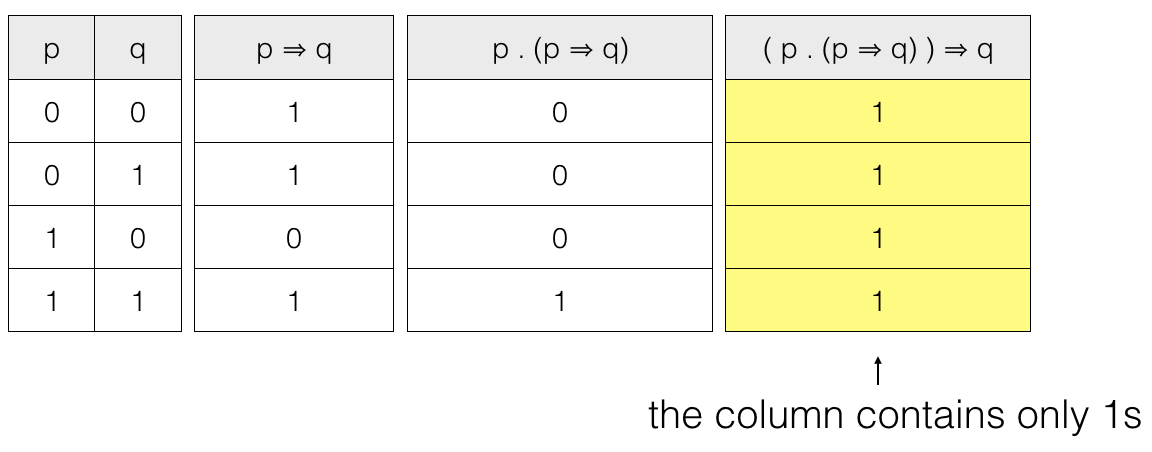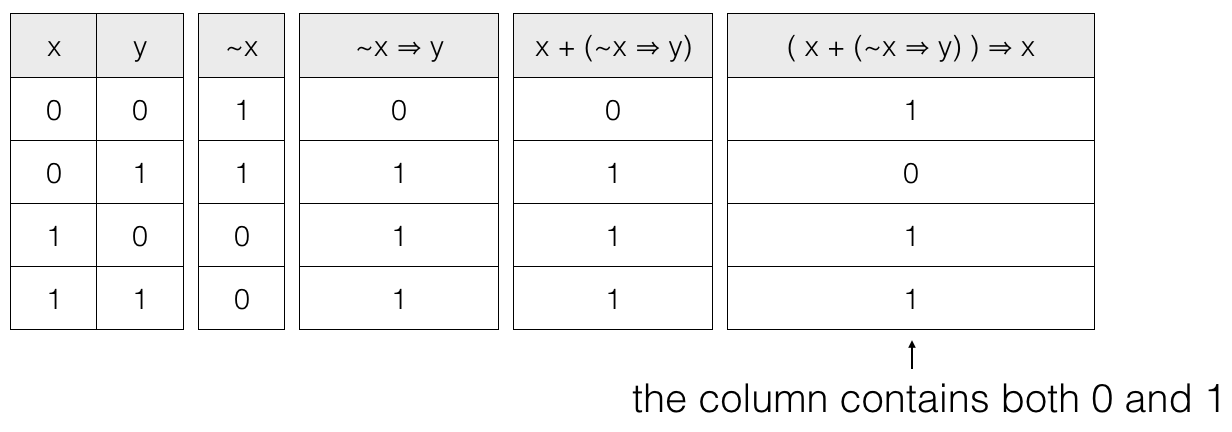# Propositional Logic Syllogism

Boolean Algebra

In this tutorial we will cover Syllogism.

## Syllogism

The logical process of finding conclusions from given propositions is called syllogism the propositions used to draw conclusion are called the premises.

We can use two methods to draw conclusion, Truth Table Method and Algebraic Method.

## Truth Table Method

In this method we draw a truth table for the premises and the conclusion.

We prepare a conditional whose antecedent is the conjunction of all the given premises and consequent is the conclusion to be drawn. If the conditional results in a tautology (i.e., all 1s or True) then the conclusion is established.

Lets see few examples to understand this :-)

## From the given premises p and p ⇒ q infer q.

So, we have two premises p and p ⇒ q. connected by an AND operator and the conclusion to be drawn is q. So we have to create a conditional
( p . (p ⇒ q) ) ⇒ q
where, . is the AND operator,
⇒ is the conditional operator

So we have to prove ( p . (p ⇒ q) ) ⇒ q is a tautology.

Truth tableSo, ( p . (p ⇒ q) ) ⇒ q is a tautology. Hence the conclusion from the given premises can be drawn.

Lets check another example.

## From the given premises x or ~x ⇒ y infer x.

so, we have two premises x and ~x ⇒ y connected by an OR operator and the conclusion to be drawn is x. So, we have to create a conditional
( x + (~x ⇒ y) ) ⇒ x
where, . is the AND operator,
+ is the OR operator,
~ is the NOT operator and
⇒ is the conditional operator

So, we have to prove ( x + (~x ⇒ y) ) ⇒ x is a tautology.

Truth tableSo, ( x + (~x ⇒ y) ) ⇒ x is not a tautology and hence the conclusion from the given premises can’t be drawn.

Now, we will talk about the Algebraic method.

## Algebraic Method

In this method we use conditional elimination to draw conclusion from given premises. We prepare a conditional whose antecedent is the conjunction of all the given premises and consequent is the conclusion to be drawn. If the conditional results in a tautology (i.e., all 1s or True) then the conclusion is established.

We substitute the conditional p ⇒ q with ~p + q
where, ~ is the NOT operator
+ is the OR operator

Lets see an example to understand this :-)

## From the given premises p and p ⇒ q infer q.

So, we have two premises p and p ⇒ q connected by an AND operator and the conclusion to be drawn is q. So, we have to create a conditional
( p . (p ⇒ q) ) ⇒ q
where, . is the AND operator
⇒ is the conditional operator

So, we have to prove ( p . (p ⇒ q) ) ⇒ q is a tautology.

 We have,( p . (p ⇒ q) ) ⇒ q = (p . (~p + q) ) ⇒ q from conditional elimination,a ⇒ b = ~a + b = (p . ~p) + (p . q) ⇒ q from distributive law,a . (b + c) = (a . b) + (a . c) = 0 + (p . q) ⇒ q from complementarity law,a . (~a) = 0 = (p . q) ⇒ q from property of 0,0 + a = a = ~(p . q) + q from conditional elimination,a ⇒ b = ~a + b = (~p + ~q) + q from de morgan’s law,~(a . b) = ~a + ~b = ~p + (~q + q) from associative law,(a + b) + c = a + (b + c) = ~p + 1 from complementarity law,(~a + a) = 1 = 1 from property of 1,~a + 1 = 1

So, ( p . (p ⇒ q) ) ⇒ q = 1 i.e., it is a tautology hence conclusion can be drawn.